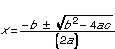# quadratic equation

quadratic equation
an equation containing a single variable of degree 2. Its general form is ax2 + bx + c = 0, where x is the variable and a, b, and c are constants (a non-zero).
[1680-90]

* * *

Algebraic equation of particular importance in optimization.

A more descriptive name is second-degree polynomial equation. Its standard form is ax2 + bx + c = 0, and its solution is given by the quadratic formulawhich guarantees two real-number solutions, one real-number solution, or two complex-number solutions, depending on whether the discriminate, b2 -4ac, is greater than, equal to, or less than 0.

* * *

in mathematics, an algebraic equation of the second degree (having one or more variables raised to the second power). Old Babylonian cuneiform texts, dating from the time of Hammurabi, show a knowledge of how to solve quadratic equations, but it appears that ancient Egyptian mathematicians did not know how to solve them. Since the time of Galileo, they have been important in the physics of accelerated motion, such as free fall in a vacuum. The general quadratic equation in one variable is ax2 + bx + c = 0, in which a, b, and c are arbitrary constants (or parameters) and a is not equal to 0. Such an equation has two roots (not necessarily distinct), as given by the quadratic formula

The discriminant b2 − 4ac gives information concerning the nature of the roots (see discriminant). If, instead of equating the above to zero, the curve ax2 + bx + c = y is plotted, it is seen that the real roots are the x coordinates of the points at which the curve crosses the x-axis. The shape of this curve in Euclidean two-dimensional space is a parabola; in Euclidean three-dimensional space it is a parabolic cylindrical surface, or paraboloid.

In two variables, the general quadratic equation is ax2 + bxy + cy2 + dx + ey + f = 0, in which a, b, c, d, e, and f are arbitrary constants and a, c ≠ 0. The discriminant (symbolized by the Greek letter delta, Δ) and the invariant (b2 − 4ac) together provide information as to the shape of the curve. The locus in Euclidean two-dimensional space of every general quadratic in two variables is a conic section or its degenerate.

More general quadratic equations, in the variables x, y, and z, lead to generation (in Euclidean three-dimensional space) of surfaces known as the quadrics, or quadric surfaces.

* * *

Universalium. 2010.

### Look at other dictionaries:

• quadratic equation — n. Algebra an equation in which the second power, or square, is the highest to which the unknown quantity is raised …   English World dictionary

• Quadratic equation — This article is about quadratic equations and solutions. For more general information about quadratic functions, see Quadratic function. For more information about quadratic polynomials, see Quadratic polynomial. In mathematics, a quadratic… …   Wikipedia

• quadratic equation — noun an equation in which the highest power of an unknown quantity is a square • Syn: ↑quadratic • Hypernyms: ↑equation * * * Math. an equation containing a single variable of degree 2. Its general form is ax2 + bx + c = 0, where x …   Useful english dictionary

• quadratic equation — UK [kwɒˌdrætɪk ɪˈkweɪʒ(ə)n] / US [kwɑˌdrætɪk ɪˈkweɪʒ(ə)n] noun [countable] Word forms quadratic equation : singular quadratic equation plural quadratic equations maths an equation in which a variable (= quantity that can represent different… …   English dictionary

• quadratic equation — equation in which one of the unknown quantities is squared (Mathematics) …   English contemporary dictionary

• quadratic equation — quad|rat|ic e|qua|tion [kwɔˌdrætık ıˈkweıʒən US kwa: ] n [Date: 1600 1700; Origin: quadratic from quadrate square (14 21 centuries), from Latin quadratus, past participle of quadrare to make square ] technical an ↑equation such ax²+by+c = z,… …   Dictionary of contemporary English

• quadratic equation — noun a polynomial equation of the second degree …   Wiktionary

• QUADRATIC EQUATION —    an equation involving the square of the unknown quantity …   The Nuttall Encyclopaedia

• quadratic equation — quad|rat|ic e|qua|tion [ kwa,drætık ı kweıʒn ] noun count in mathematics, an EQUATION in which a VARIABLE (=quantity which can represent different amounts) is multiplied by itself once only …   Usage of the words and phrases in modern English

• quadratic equation — noun (C) technical an equation such as x/^2+bx+c=y, which includes numbers or quantities multiplied by themselves …   Longman dictionary of contemporary English

### Share the article and excerpts

##### Direct link
Do a right-click on the link above
and select “Copy Link”